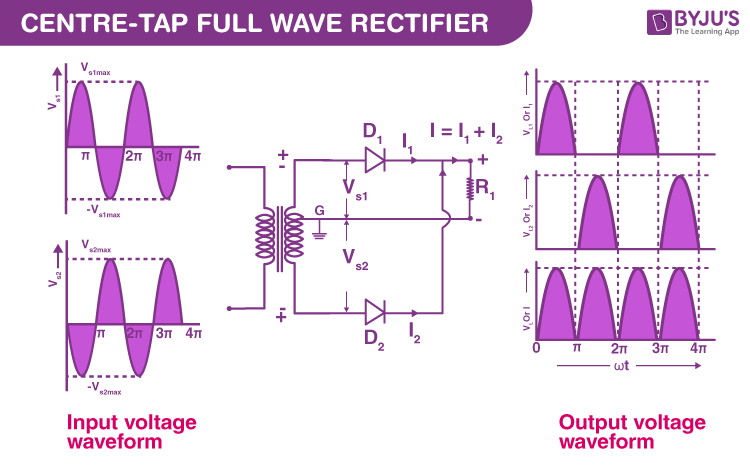# Full Wave Rectifier

Electric circuits that convert AC to DC are known as rectifiers. Rectifiers are classified into two types as Half Wave Rectifiers and Full Wave Rectifiers. Significant power is lost while using a half-wave rectifier and is not feasible for applications that need a smooth and steady supply. For a more smooth and steady supply, we use the full wave rectifiers. In this article, we will be looking into the working and characteristics of a full wave rectifier.

## Defining Full Wave Rectifiers

A full wave rectifier is defined as a rectifier that converts the complete cycle of alternating current into pulsating DC.

Unlike halfwave rectifiers that utilize only the halfwave of the input AC cycle, full wave rectifiers utilize the full cycle. The lower efficiency of the half wave rectifier can be overcome by the full wave rectifier.

### Full Wave Rectifier Circuit

The circuit of the full wave rectifier can be constructed in two ways. The first method uses a centre tapped transformer and two diodes. This arrangement is known as a centre tapped full wave rectifier. The second method uses a standard transformer with four diodes arranged as a bridge. This is known as a bridge rectifier. In the next section, we will restrict the discussion to the centre tapped full wave rectifier only. You can read our article on bridge rectifier to learn the construction and working of bridge rectifier in detail.The circuit of the full wave rectifier consists of a step-down transformer and two diodes that are connected and centre tapped. The output voltage is obtained across the connected load resistor.

### Working of Full Wave Rectifier

The input AC supplied to the full wave rectifier is very high. The step-down transformer in the rectifier circuit converts the high voltage AC into low voltage AC. The anode of the centre tapped diodes is connected to the transformer’s secondary winding and connected to the load resistor. During the positive half cycle of the alternating current, the top half of the secondary winding becomes positive while the second half of the secondary winding becomes negative.

During the positive half cycle, diode D1 is forward biased as it is connected to the top of the secondary winding while diode D2 is reverse biased as it is connected to the bottom of the secondary winding. Due to this, diode D1 will conduct acting as a short circuit and D2 will not conduct acting as an open circuit

During the negative half cycle, the diode D1 is reverse biased and the diode D2 is forward biased because the top half of the secondary circuit becomes negative and the bottom half of the circuit becomes positive. Thus in a full wave rectifiers, DC voltage is obtained for both positive and negative half cycle.

## Full Wave Rectifier Formula

### Peak Inverse Voltage

Peak inverse voltage is the maximum voltage a diode can withstand in the reverse-biased direction before breakdown. The peak inverse voltage of the full-wave rectifier is double that of a half-wave rectifier. The PIV across D1 and D2 is 2Vmax.

### DC Output Voltage

The following formula gives the average value of the DC output voltage:

$$\begin{array}{l}V_{dc}=I_{av}R_{L}=\frac{2}{\pi}I_{max}R_{L}\end{array}$$

### RMS Value of Current

The RMS value of the current can be calculated using the following formula:

$$\begin{array}{l}I_{rms}=\frac{I_{max}}{\sqrt{2}}\end{array}$$

### Form Factor

The form factor of the full wave rectifier is calculated using the formula:

$$\begin{array}{l}K_{f}=\frac{RMS\,value\,of\,current}{Average\,value\,of\,current}=\frac{I_{rms}}{I_{dc}}=\frac{I_{max}/\sqrt{2}}{2I_{max}/{\pi}}=\frac{\pi }{2\sqrt{2}}=1.11\end{array}$$

### Peak Factor

The following formula gives the peak factor of the full wave rectifier:

$$\begin{array}{l}K_{p}=\frac{Peak\,value\,of\,current}{RMS\,value\,of\,current}=\frac{I_{max}}{I_{max}/\sqrt{2}}=\sqrt{2}\end{array}$$

### Rectification Efficiency

The rectification efficiency of the full-wave rectifier can be obtained using the following formula:

$$\begin{array}{l}\eta=\frac{DC\,Output\,Power}{AC\,Output\,Power} \end{array}$$

The efficiency of the full wave rectifiers is 81.2%.

## Advantages of Full Wave Rectifier

• The rectification efficiency of full wave rectifiers is double that of half wave rectifiers. The efficiency of half wave rectifiers is 40.6% while the rectification efficiency of full wave rectifiers is 81.2%.
• The ripple factor in full wave rectifiers is low hence a simple filter is required. The value of ripple factor in full wave rectifier is 0.482 while in half wave rectifier it is about 1.21.
• The output voltage and the output power obtained in full wave rectifiers are higher than that obtained using half wave rectifiers.

The only disadvantage of the full wave rectifier is that they need more circuit elements than the half wave rectifier which makes, making it costlier.

## Frequently Asked Questions – FAQs

Q1

### What is a full wave rectifier?

Full wave rectifiers convert both polarities of the input AC waveform to pulsating DC.

Q2

### Why do we use a capacitor in full wave rectifier circuit?

A capacitor is used in the circuit to reduce the ripple factor.

Q3

### What is a centre tapped full wave rectifier?

A centre tapped full wave rectifier is a type of rectifier that uses a centre tapped transformer and two diodes to convert the complete AC signal into DC signal.

Q4

### Where is a full wave rectifier used?

A full wave rectifier is used in signal modulation and in electric welding.

Q5

### What are the disadvantages of full wave rectifiers?

The full wave rectifiers are not suitable to use when a small voltage is required to be rectified. This is because, in a fullwave circuit, two diodes are connected in series and offer double voltage drop due to internal resistances.

Stay tuned to BYJU’S and Fall in Love with Learning!